## Getting Started: QP Solver

Consider a small illustrative example. Suppose you want to minimize a two-variable quadratic function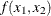on the nonnegative quadrant, subject to two constraints: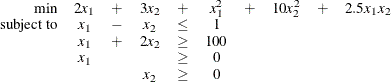To use the OPTMODEL procedure, it is not necessary to fit this problem into the general QP formulation mentioned in the section Overview: QP Solver and to compute the corresponding parameters. However, since these parameters are closely related to the data set that is used by the OPTQP procedure and has a quadratic programming system (QPS) format, you can compute these parameters as follows. The linear objective function coefficients, vector of right-hand sides, and lower and upper bounds are identified immediately as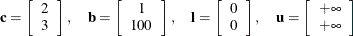Carefully construct the quadratic matrix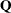. Observe that you can use symmetry to separate the main-diagonal and off-diagonal elements: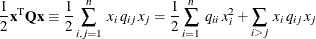The first expression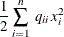sums the main-diagonal elements. Thus, in this case you have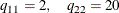Notice that the main-diagonal values are doubled in order to accommodate the 1/2 factor. Now the second term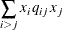sums the off-diagonal elements in the strict lower triangular part of the matrix. The only off-diagonal (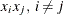) term in the objective function is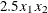, so you have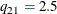Notice that you do not need to specify the upper triangular part of the quadratic matrix.

Finally, the matrix of constraints is as follows: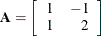The following OPTMODEL program formulates the preceding problem in a manner that is very close to the mathematical specification of the given problem:

/* getting started */
proc optmodel;
var x1 >= 0; /* declare nonnegative variable x1 */
var x2 >= 0; /* declare nonnegative variable x2 */

/* objective: quadratic function f(x1, x2) */
minimize f =
/* the linear objective function coefficients */
2 * x1 + 3 * x2 +

x1 * x1 + 2.5 * x1 * x2 + 10 * x2 * x2;

/* subject to the following constraints */
con r1: x1 - x2 <= 1;
con r2: x1 + 2 * x2 >= 100;

/* specify iterative interior point algorithm (QP)
* in the SOLVE statement */
solve with qp;

/* print the optimal solution */
print x1 x2;
save qps qpsdata;
quit;


The with qp clause in the SOLVE statement invokes the QP solver to solve the problem. The output is shown in Figure 9.2.

Figure 9.2: Summaries and Optimal Solution

The OPTMODEL Procedure

Problem Summary
Objective Sense Minimization
Objective Function f

Number of Variables 2
Bounded Above 0
Bounded Below 2
Bounded Below and Above 0
Free 0
Fixed 0

Number of Constraints 2
Linear LE (<=) 1
Linear EQ (=) 0
Linear GE (>=) 1
Linear Range 0

Constraint Coefficients 4

Performance Information
Execution Mode Single-Machine

Solution Summary
Solver QP
Algorithm Interior Point
Objective Function f
Solution Status Optimal
Objective Value 15018

Primal Infeasibility 2.110419E-16
Dual Infeasibility 8.727374E-15
Bound Infeasibility 0
Duality Gap 3.633377E-16
Complementarity 0

Iterations 6
Presolve Time 0.00
Solution Time 0.14

x1 x2
34 33

In this example, the SAVE QPS statement is used to save the QP problem in the QPS-format data set qpsdata, shown in Figure 9.3. The data set is consistent with the parameters of general quadratic programming previously computed. Also, the data set can be used as input to the OPTQP procedure.

Figure 9.3: QPS-Format Data Set

Obs FIELD1 FIELD2 FIELD3 FIELD4 FIELD5 FIELD6
1 NAME   qpsdata .   .
2 ROWS     .   .
3 N f   .   .
4 L r1   .   .
5 G r2   .   .
6 COLUMNS     .   .
7   x1 f 2.0 r1 1
8   x1 r2 1.0   .
9   x2 f 3.0 r1 -1
10   x2 r2 2.0   .
11 RHS     .   .
12   .RHS. r1 1.0   .
13   .RHS. r2 100.0   .
14 QSECTION     .   .
15   x1 x1 2.0   .
16   x1 x2 2.5   .
17   x2 x2 20.0   .
18 ENDATA     .   .